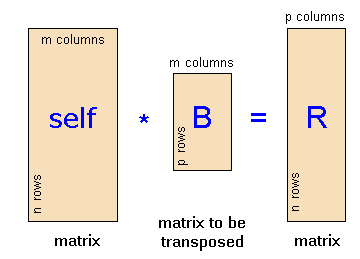The SDL Component Suite is an industry leading collection of components supporting scientific and engineering computing. Please visit the SDL Web site for more information....## MultiplyTransposed

 Unit: SDL_matrix Class: Declaration: function MultiplyTransposed (MatB, MatRes: TMatrix): boolean; { class TMatrix } function MultiplyTransposed (MatB, MatRes: TIntMatrix): boolean; { class TIntMatrix }

The function MultiplyTransposed calculates the product of matrix self with the matrix MatB after transposing it. The results are stored in MatRes. The function returns the value TRUE, if the multiplication has been carried out successfully.

 Hint: The multiplication of two matrices is only possible if the number of columns of the first matrix (self ) equals the number of rows of the second matrix (MatBT). The resulting matrix MatRes is automatically resized to the appropriate dimensions. The following figure illustrates the context:Example: The statement MatA.Multiply (MatB, MatR) multiplies the matrix MatA with the transpose of MatB and stores the result in MatR.

Last Update: 2016-Jun-28